#Function Repository Resource:

# AstroDistance

Computes the distance between astronomical entities

Contributed by: Jeff Bryant
 ResourceFunction["AstroDistance"][entity1,entity2] computes the distance between entity1 and entity2 for the current date and time. ResourceFunction["AstroDistance"][entity1,entity2,date] computes the distance between entity1 and entity2 for the specified date. ResourceFunction["AstroDistance"][{entity1,…,entityn}] computes the distance between entity1 and entityn, through all the intermediate entityi, for the current date and time. ResourceFunction["AstroDistance"][{entity1,…,entityn},date] computes the total distance from entity1 to entityn, through all the intermediate entityi, for the specified date.

## Details and Options

An internet connection is required.
date must be a DateObject.
Shorter dates can be used: DateObject[{y}] is equivalent to DateObject[{y,1,1,0,0,0}], DateObject[{y,m}] to DateObject[{y,m,1,0,0,0}], etc.
The coordinates of all entities must be known or a missing result will be returned.
Distances are all measured from center to center and along a straight line.
Distances are returned in astronomical units by default.
Distances to extra‐solar system objects are based on light‐travel distances for a fixed epoch and ignore effects such as proper motion.

## Examples

### Basic Examples

Compute the current distance between Mars and Jupiter:

 In:=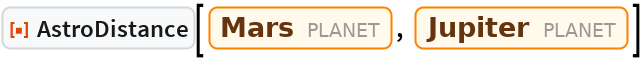Out=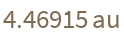Compute the distance between Mars and Jupiter on a specified date:

 In:=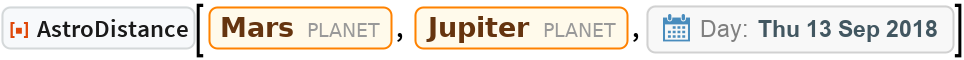Out=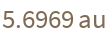Compute the current total distance between entities:

 In:=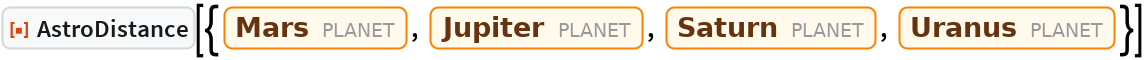Out=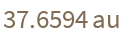Compute the total distance between entities on a specified date:

 In:=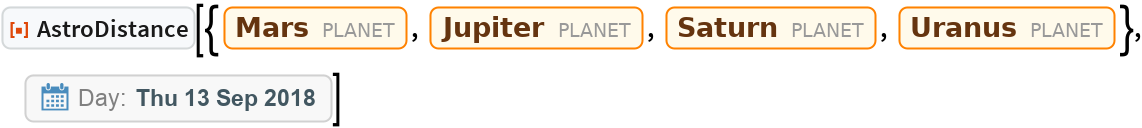Out=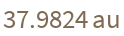### Scope

Entities can be in different domains:

 In:=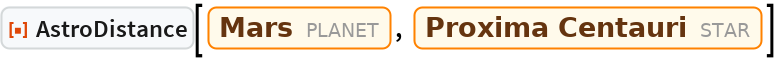Out=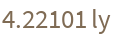In:=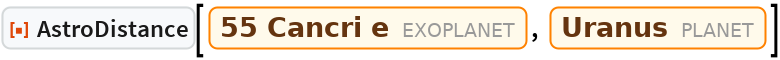Out=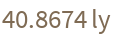Results are automatically given in appropriate units by default:

 In:=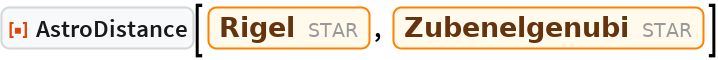Out=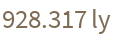### Options

Target units can be specified:

 In:=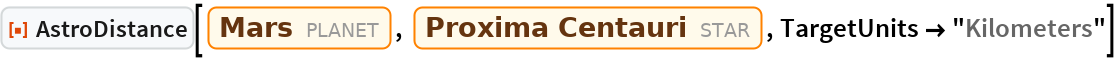Out=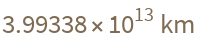## Requirements

Wolfram Language 11.3 (March 2018) or above